# A Different Way to Look at Partitions.

Quite some time ago I watched the movie 'The Man Who Knew Infinity' which most of you must be knowing is a real life story based on a very respectable Indian mathematician Srinivasa Ramanujan. I had not even heard of the partition until it was mentioned in the movie. So after that I looked up on the internet about partition theory and went on my own expedition to find the number of partitions of a number. It's not an amazing new formula to magically output value of number of partitions for a given input, rather it is a long method which incorporates visualization (much similar to Young's diagrams and Ferrer's diagrams) to find the number of partitions of a given positive integer. Now without any further ado, here is what I thought about it.

Let's suppose that one mushroom represents the number $1$, vertical stacking adds the ones and horizontal stacking of vertical stacks adds the numbers. For eg. $3+2+1$ will be represented as :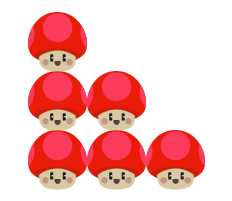$[$Note that $3+2+1$ is same as $3+1+2$ or $2+1+3$ $]$

The number $4$ can be represented as 'Mushroom Diagram' in following ways -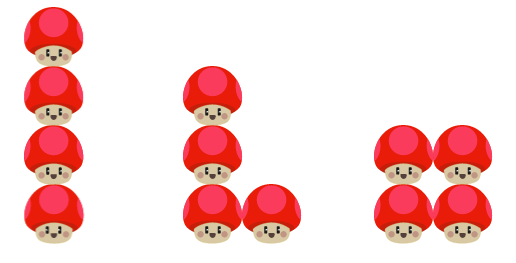Let's add another notation here, let $P_{n}$ denote the number of partitions of integer $n$.Now, we will consider $1+1+1+1.... n$ times as the $Base Partition$ and we will do some operations on this $Base Partition$ to get our desired results. Note that $Base Partition$ is the most $wide$ partition out of all.

Firstly we take $m$ mushrooms from lined up $n$ mushrooms and now what we have left is $n-m$ mushrooms in a row and the $m$ mushrooms we picked up. Now we have to stack up these $m$ mushrooms on top of $n-m$ mushrooms, somewhat like this-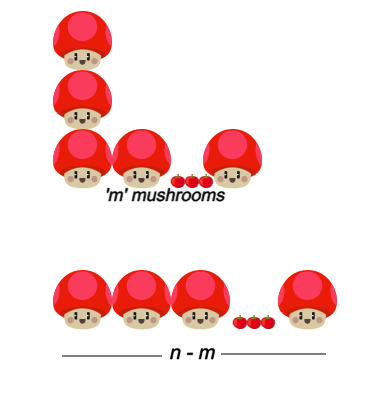Now things start to get a little bit messy because of the two possibilities viz. -

i) $n-m > m$

ii) $n-m < m$

## 1.$n-m > m$

This is an easy one as the number of ways the $m$ mushrooms can be stacked upon $n-m$ mushrooms is equal to the number of partitions of $m$ because even the Base Partition of $m$ mushrooms would fit on the $n-m$ mushrooms. So, the number of ways to stack $m$ mushrooms if $n-m>m$ is $P_{m}$. For eg. for $n=6$ and $m=2$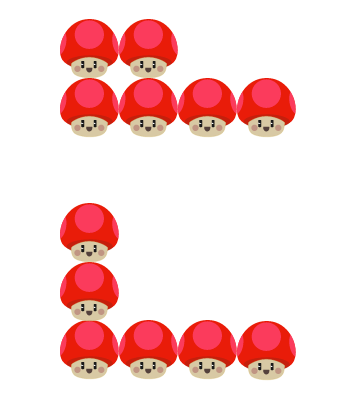## 2.$n-m

In this case we would not be able to stack all the partitions of $m$ on the $n-m$ mushrooms lined up because the Base Partition along with some other partitions would not fit. So we will have to subtract some cases to get the actual number of ways. If $n$ is even the maximum vertical stack of mushrooms left un-stacked would be $2,4,6,8.....$ depending on value of $m$. And in case of odd $n$ the number of mushrooms left unstacked would be $1,3,5,7.....$ depending on value of $m$. For eg. if $n=6$ and $m=4$ the maximum number of mushrooms left out would be 2.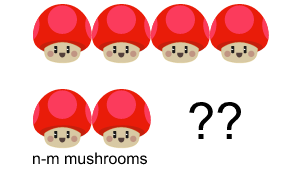And we will have to subtract the cases where taken mushrooms are not fully stacked as it would lead to repetition of cases. Let's introduce another variable $t$ which represents the maximum number of mushrooms left out which can also be written in other form $2m-n$. Now, we can find the number of cases to be subtracted from $P_{m}$ to get the desired cases. We have to arrange $m$ mushrooms so we will have to subtract those cases where the number of horizontal rows is more than $n-m$. In order to achieve this we will first lay the mushrooms in horizontal row and work our way backwards by picking up one at a time and then arranging them too.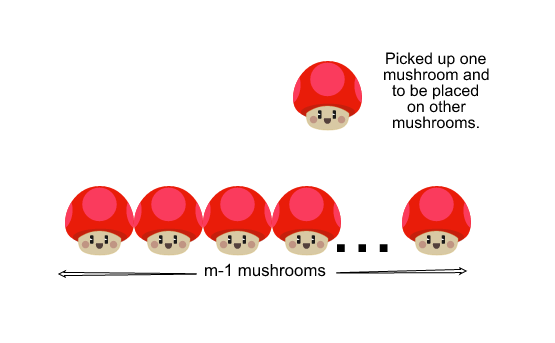So the number of discarded cases would be $P_{0} + P_{1} + P_{2} ...$ until the point we pick up less mushrooms than we have bases to arrange them on. And we will be faced by the same dilemma because of which we divided the case into two parts.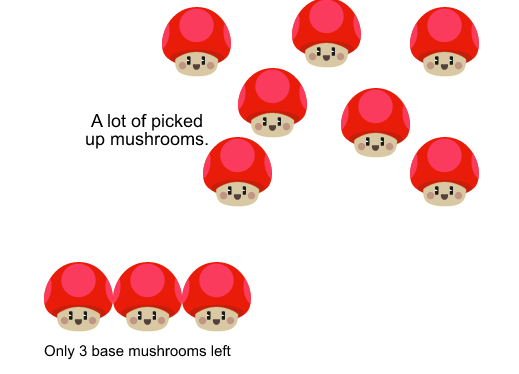And we will have to keep on doing the things we did until we reach a point where we can easily stack mushrooms.We can also find approximate value by cutting off at any step and simply assuming number of discarded cases to be $P_{1} + P_{2} .... P_{t}$ but remember the more times you will apply this algorithm, more close to the answer you will be.

P.S. If you could find the number of partitions of integer $n$ which only has a specific number of bases, say $x$, it would make the problem a lot easier to solve. Somewhat like this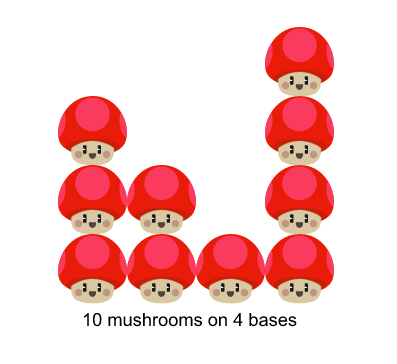I know that this is not the best way of finding the number of partitions and I would really welcome your comments to improvise this method.Thank you for any suggestions.Note by Jayesh Swami
4 years, 6 months ago

This discussion board is a place to discuss our Daily Challenges and the math and science related to those challenges. Explanations are more than just a solution — they should explain the steps and thinking strategies that you used to obtain the solution. Comments should further the discussion of math and science.

When posting on Brilliant:

• Use the emojis to react to an explanation, whether you're congratulating a job well done , or just really confused .
• Ask specific questions about the challenge or the steps in somebody's explanation. Well-posed questions can add a lot to the discussion, but posting "I don't understand!" doesn't help anyone.
• Try to contribute something new to the discussion, whether it is an extension, generalization or other idea related to the challenge.

MarkdownAppears as
*italics* or _italics_ italics
**bold** or __bold__ bold
- bulleted- list
• bulleted
• list
1. numbered2. list
1. numbered
2. list
Note: you must add a full line of space before and after lists for them to show up correctly
paragraph 1paragraph 2

paragraph 1

paragraph 2

[example link](https://brilliant.org)example link
> This is a quote
This is a quote
    # I indented these lines
# 4 spaces, and now they show
# up as a code block.

print "hello world"
# I indented these lines
# 4 spaces, and now they show
# up as a code block.

print "hello world"
MathAppears as
Remember to wrap math in $$ ... $$ or $ ... $ to ensure proper formatting.
2 \times 3 $2 \times 3$
2^{34} $2^{34}$
a_{i-1} $a_{i-1}$
\frac{2}{3} $\frac{2}{3}$
\sqrt{2} $\sqrt{2}$
\sum_{i=1}^3 $\sum_{i=1}^3$
\sin \theta $\sin \theta$
\boxed{123} $\boxed{123}$